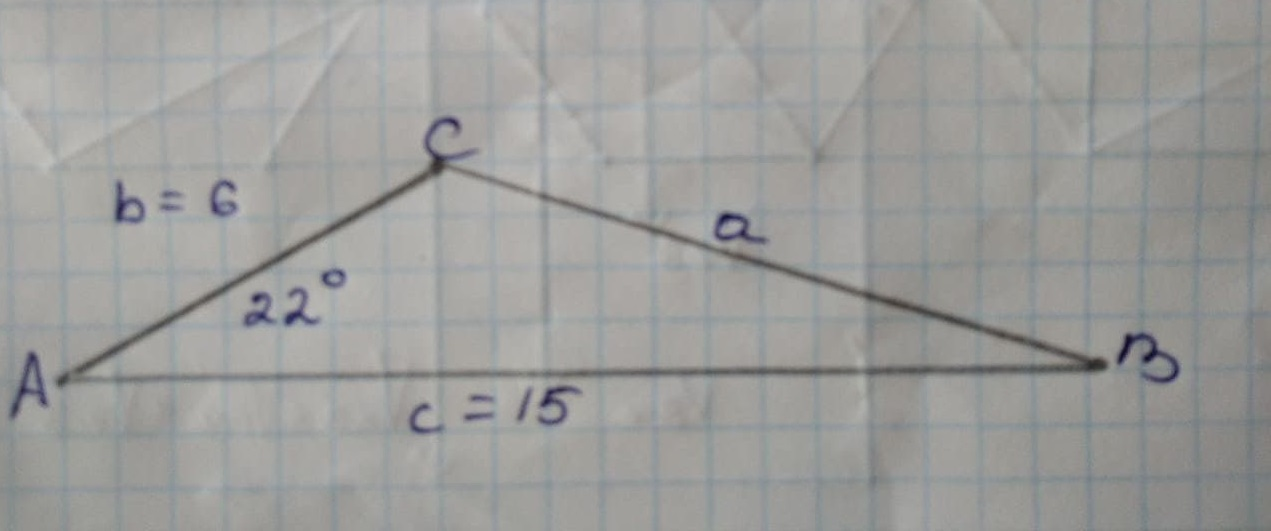## Use the Law of Cosines to solve the triangles.

TrigonometryUse the Law of Cosines to solve the triangles.2 years ago
Use the Law of Cosines to solve the triangles. Round lengths to the nearest tenth and angle measures to the nearest degree. PLEASE solve both of these triangles. Thank you!using Cosine rule, cosA =(b^(2)+c^(2)-a^(2))/(2bc) -> cos(22)=((6)^(2)+(15)^(2)-a^(2))/(2(6)(15)) -> 0/9272=(36+225-a^(2))/(180) -> 166.893=261-a^(2) -> a^(2)=94.1069 -> a=9.7009 -> ~ 10 using Sine rule, (sin A)/(a)=(sinB)/(b) -> (sin(22))/(10)=(sinB)/(6) -> sin B=(6sin(22))/(10) -> B~ 13° using angle sum property of a triangle, C = 180- (A+B) = 180 - (22 + 13) = 145° Therefore, a=10,B=13°,C=145°